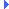FD.io VPP  v18.07.1-13-g909ba93 Vector Packet Processing
xy.h File ReferenceInclude dependency graph for xy.h:

Go to the source code of this file.

## Macros

#define xy_x(x)   __real__ (x)

#define xy_y(x)   __imag__ (x)

#define xy_x_unit_vector   (1)

#define xy_y_unit_vector   (1I)

## Typedefs

typedef double xy_float_t

typedef __complex__ double xy_t

typedef __complex__ int ixy_t

typedef __complex__ char i8xy_t

typedef __complex__ short i16xy_t

typedef __complex__ int i32xy_t

## Macro Definition Documentation

 #define xy_x ( x ) __real__ (x)

Definition at line 41 of file xy.h.

 #define xy_x_unit_vector   (1)

Definition at line 45 of file xy.h.

 #define xy_y ( x ) __imag__ (x)

Definition at line 42 of file xy.h.

 #define xy_y_unit_vector   (1I)

Definition at line 46 of file xy.h.

## Typedef Documentation

 typedef __complex__ short i16xy_t

Definition at line 37 of file xy.h.

 typedef __complex__ int i32xy_t

Definition at line 38 of file xy.h.

 typedef __complex__ char i8xy_t

Definition at line 36 of file xy.h.

 typedef __complex__ int ixy_t

Definition at line 34 of file xy.h.

 typedef double xy_float_t

Definition at line 32 of file xy.h.

 typedef __complex__ double xy_t

Definition at line 33 of file xy.h.